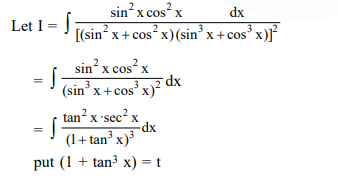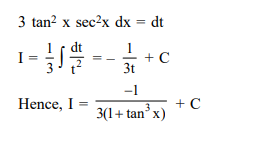# The integral

Question:

The integral $\int \frac{\sin ^{2} x \cos ^{2} x}{\left(\sin ^{5} x+\cos ^{3} x \sin ^{2} x+\sin ^{3} x \cos ^{2} x+\cos ^{5} x\right)^{2}} d x$ is equal to (where C is a constant of integration)

1. $\frac{-1}{3\left(1+\tan ^{3} x\right)}+C$

2. $\frac{1}{1+\cot ^{3} x}+C$

3. $\frac{-1}{1+\cot ^{3} x}+C$

4. $\frac{1}{3\left(1+\tan ^{3} x\right)}+C$

Correct Option: 1

Solution: